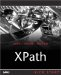# Using Node Functions

The XPath 2.0 node functions let you handle nodes, and here's an overview of these functions:

• fn: name returns the name of the specified node as an xs:string value.

• fn:local-name returns the local name of the specified node as an xs:NCName value.

• fn:namespace-uri returns the namespace URI as an xs:string value for a given node.

• fn:number returns the value of the specified item converted to an xs:double value.

• fn:lang returns a Boolean value, depending on whether the language of the context node (defined with the xml:lang attribute) matches the language passed to this function.

• fn:root returns the root of the tree to which the node argument belongs.

There's also a set of op functions (corresponding to operators we already know about) that we won't cover in detail:

• op:is-same-node returns true if the two arguments have the same identity. Backs up the is operator.

• op:node-before indicates whether one node appears before another node in document order. Backs up the << operator.

• op:node-after indicates whether one node appears after another node in document order. Backs up the >> operator.

We'll start covering the XPath 2.0 node functions in depth now.

#### The fn:name Function

This function returns the name of a node as an xs:string value, which satisfies the restrictions on xs:QName values:

` `
` fn:name() as xs:string fn:name(  \$srcval  as node?) as xs:string `

If you don't pass any argument to this function, it uses the context node. Also, if the node has no name (as is the case for a document node, a comment, a text node, or a namespace node having no name), you get an empty string return. If the node you're working with has a namespace prefix, you'll get the node's name in the form prefix:local-name .

You can see an example using this function in ch12_01.xsl (Listing 12.1), where we use this function to determine the name of a <planet> node in our planetary data document.

##### Listing 12.1 Using fn:name ( ch12_01.xsl )
` <xsl:stylesheet version="2.0"     xmlns:xsl="http://www.w3.org/1999/XSL/Transform"     xmlns:xs="http://www.w3.org/2001/XMLSchema">  <xsl:template match="/planets/planet">   <xsl:value-of select="name()"/>   </xsl:template>  <xsl:template match="/planets/planet  /planets/planet">     </xsl:template> </xsl:stylesheet> `

And here's the result:

` `
` <?xml version="1.0" encoding="UTF-8"?> planet `

#### The fn:local-name Function

This function is just the same as fn:name , except that it returns the node's local name only. Here's how you use it:

` `
` fn:local-name() as xs:string fn:local-name(\$srcval as node?) as xs:string `

If you don't supply an argument, this function uses the context node.

#### The fn:namespace-uri Function

This function returns the namespace URI of a node as an xs:string value:

` `
` fn:namespace-uri() as xs:string fn:namespace-uri(\$srcval as node?) as xs:string `

If you don't pass it any arguments, this function uses the context node. If the node has no namespace URI, you'll get an empty string return value.

#### The fn:number Function

The fn:number function treats the value of a node as a number and returns that number as an xs:double value. Here's how you use this function:

` `
` fn:number() as xs:double fn:number(  \$srcval  as item?) as xs:double `

Note that if the value of the node cannot be converted to an xs:double value (see the XPath specification for the exact details on how this conversion is made), the NaN (Not a Number) value is returned.

#### The fn:lang Function

This function lets you check the language of the context nodegiven by the xml:lang attribute:

` `
` fn:lang(  \$testlang  as xs:string) as xs:boolean `

The fn:lang function returns true if the language of the context node is the same asor a sublanguage ofthe language given by \$testlang , and false otherwise . Note that if there is no context node, this function returns false .

For example, say that we add an xml:lang attribute to our planetary data document:

` `
` <?xml version="1.0" encoding="UTF-8"?> <planets>  <planet xml:lang="en-us">  <name>Mercury</name>         <mass units="(Earth = 1)">.0553</mass>         <day units="days">58.65</day>         <radius units="miles">1516</radius>         <density units="(Earth = 1)">.983</density>         <distance units="million miles">43.4</distance>         <!--At perihelion-->     </planet>         .         .         . `

Now we can check the language used in this example as you see in ch12_02.xsl (Listing 12.2).

##### Listing 12.2 Using fn:lang ( ch12_02.xsl )
` <xsl:stylesheet version="2.0"     xmlns:xsl="http://www.w3.org/1999/XSL/Transform"     xmlns:xs="http://www.w3.org/2001/XMLSchema">  <xsl:template match="/planets/planet">   <xsl:value-of select="if(lang('en-us'))   then 'Correct.'   else 'Richtig.'"/>   </xsl:template>  <xsl:template match="/planets/planet  /planets/planet">     </xsl:template> </xsl:stylesheet> `

And here's the result:

` `
` <?xml version="1.0" encoding="UTF-8"?> Correct. `

#### The fn:root Function

The fn:root function returns the root of the tree to which the context node, or the node you pass, belongs. Here's how you use this function:

` `
` fn:root() as node fn:root(  \$srcval  as node) as node `

Note that the root node that this function returns is usually, but doesn't have to be, a document node. If you don't pass a node at all, the context node is used.

Here's an examplein this case, we'll select the first planet in our planetary data document, Mercury, and then access the name of the second planet using the expression root()/planets/planet/name , as you see in ch12_03.xsl (Listing 12.3).

##### Listing 12.3 Using fn:root ( ch12_03.xsl )
` <xsl:stylesheet version="2.0"     xmlns:xsl="http://www.w3.org/1999/XSL/Transform"     xmlns:xs="http://www.w3.org/2001/XMLSchema">  <xsl:template match="/planets/planet">   <xsl:value-of select="root()/planets/planet/name"/>   </xsl:template>  <xsl:template match="/planets/planet  /planets/planet">     </xsl:template> </xsl:stylesheet> `

And here's the resultas you can see, we were indeed able to extract the name of the second planet this way:

` `
` <?xml version="1.0" encoding="UTF-8"?> Venus `

That takes care of the XPath 2.0 node functions; we're going to turn to the functions that handle sequences next .XPath Kick Start: Navigating XML with XPath 1.0 and 2.0
ISBN: 0672324113
EAN: 2147483647
Year: 2002
Pages: 131

Similar book on Amazon